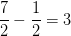# SSAT Upper Level Math : How to find the next term in an arithmetic sequence

## Example Questions

← Previous 1

### Example Question #51 : Sequences And Series

What is the value of x in the sequence below?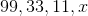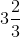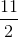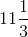Explanation:

In this sequence, each subsequent number is equal to one third of the preceding number.

One third of 11 is equal to: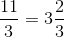Therefore, the correct answer is:### Example Question #52 : Sequences And Series

Find the next term of the arithmetic sequence: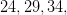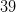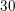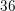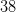Explanation:

The common difference for this sequence is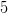. To find the next number in the sequence, addto the last given number.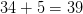### Example Question #51 : Sequences And Series

Find the next term of this arithmetic sequence: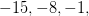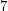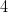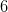Explanation:

The common difference for this sequence is. Add this to the last given term to find the next one.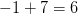### Example Question #1 : How To Find The Next Term In An Arithmetic Sequence

Find the next term of the arithmetic sequence: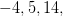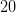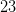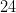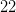Explanation:

The common difference is. Add this to the last given term to find the next term.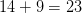### Example Question #31 : Arithmetic Sequences

Find the next term of the arithmetic sequence: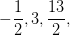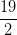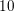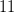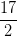Explanation:

The common difference is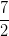. Add this to the last given term to find the next term.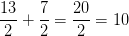### Example Question #1 : How To Find The Next Term In An Arithmetic Sequence

Find the next term of the arithmetic sequence: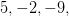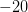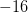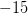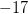Explanation:

The common difference is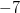. Add this to the last given term to find the next term.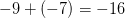### Example Question #12 : Nth Term Of An Arithmetic Sequence

Find the next term of the arithmetic sequence: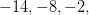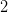Explanation:

The common difference is. Add this to the last given term to find the next term.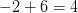### Example Question #1 : How To Find The Next Term In An Arithmetic Sequence

Find the next term of the arithmetic sequence: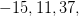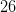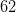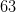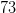Explanation:

The common difference is. Add this to the last given term to find the next term.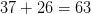### Example Question #35 : Arithmetic Sequences

Find the next term of the arithmetic sequence: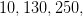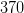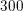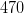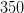Explanation:

The common difference is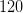. Add this to the last given term to find the next term.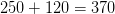### Example Question #21 : Nth Term Of An Arithmetic Sequence

Find the next term in the following arithmetic sequence: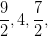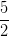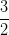The common difference for this arithmetic sequence is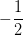. To find the next term in the sequence, subtract that value from the last given value.